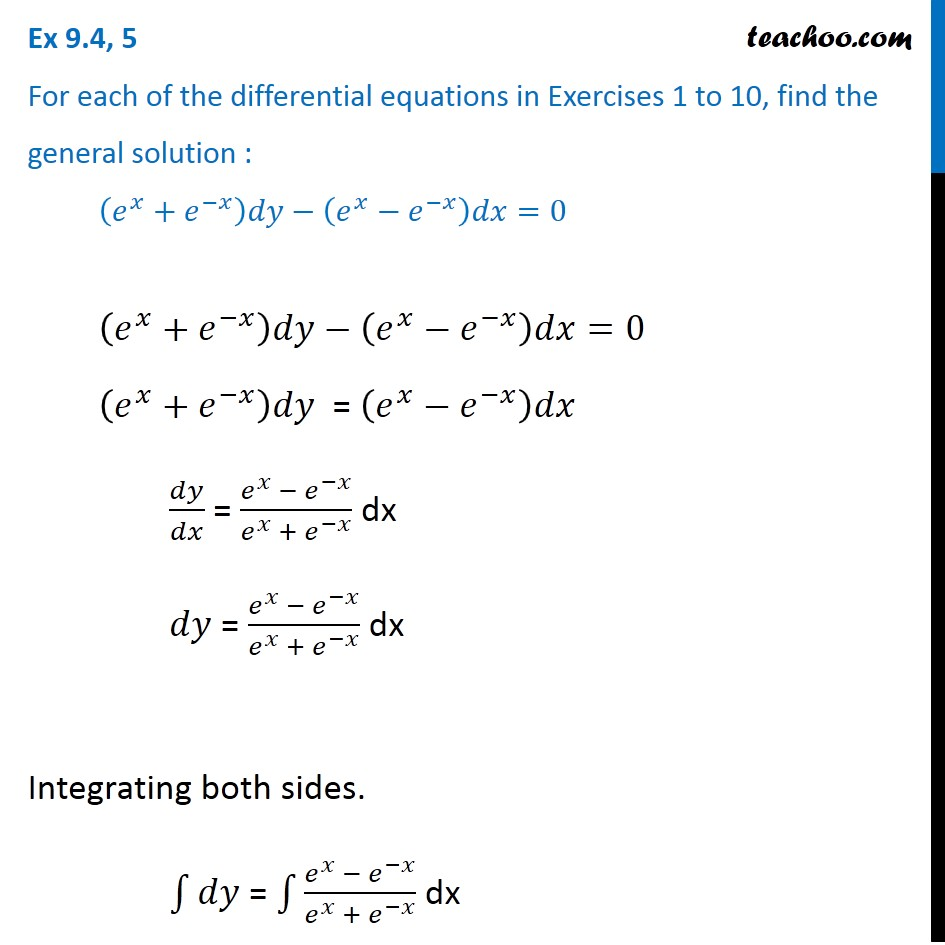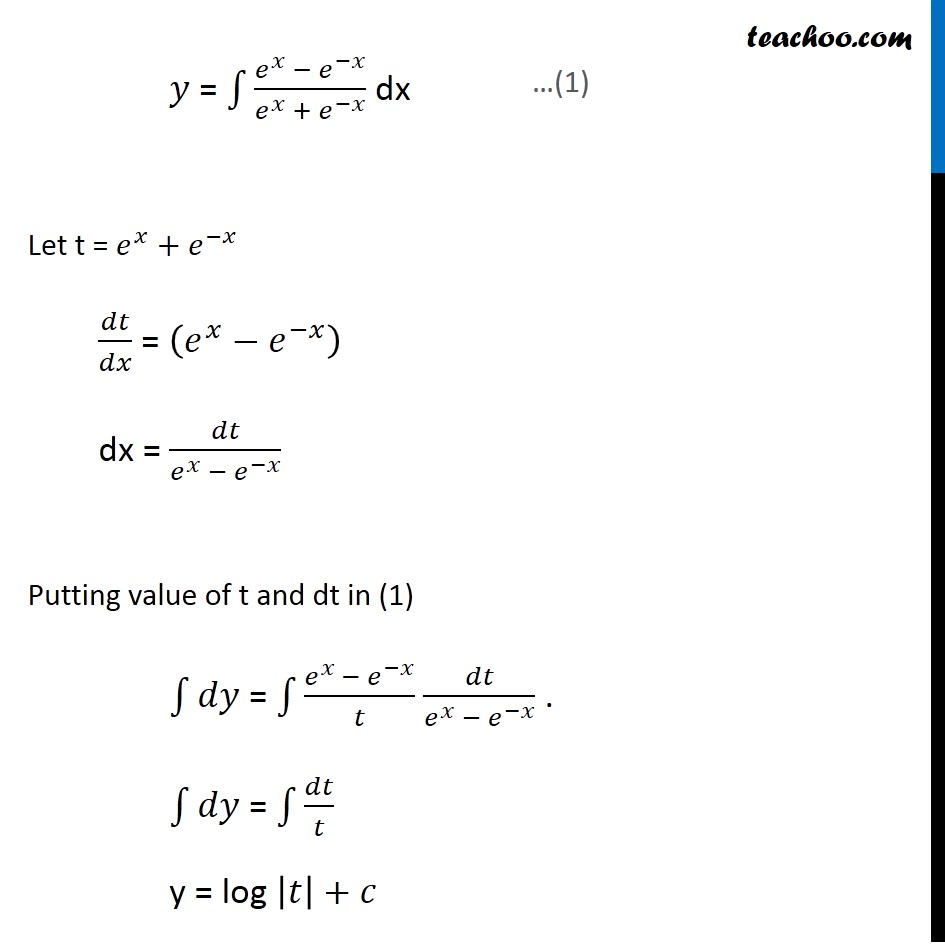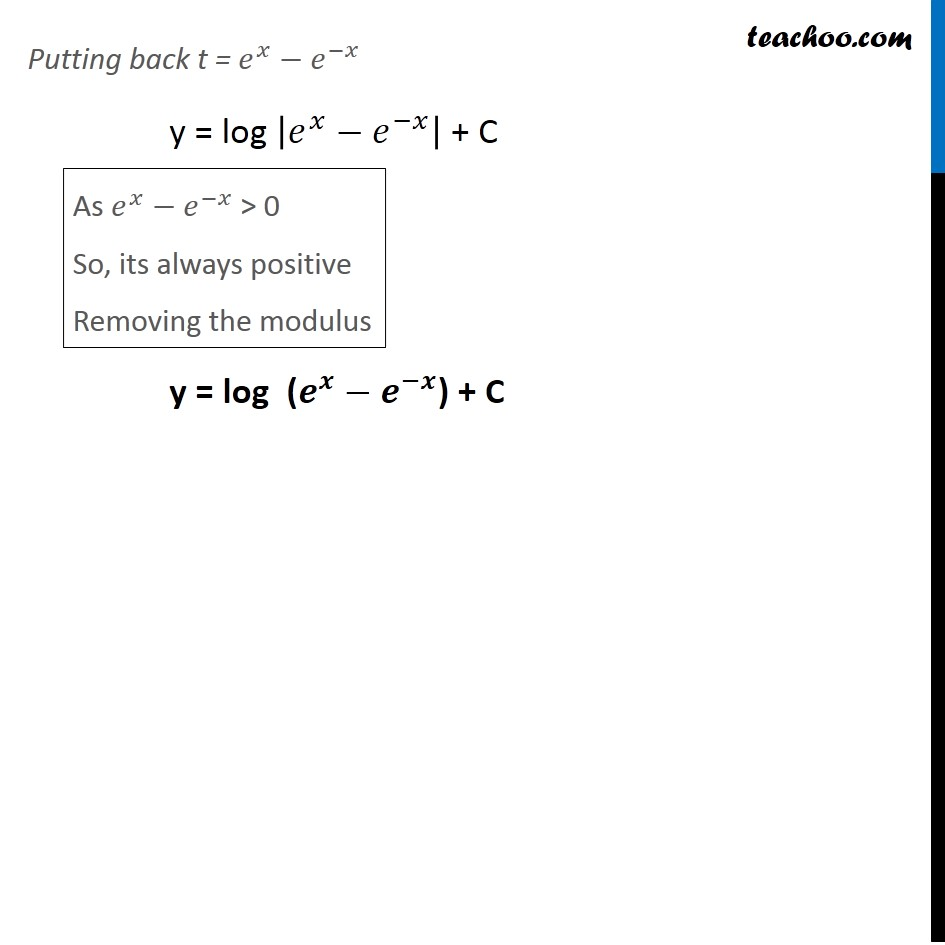Ex 9.3

Chapter 9 Class 12 Differential Equations
Serial order wiseLearn in your speed, with individual attention - Teachoo Maths 1-on-1 Class

### Transcript

Ex 9.3, 5 For each of the differential equations in Exercises 1 to 10, find the general solution : (𝑒^𝑥+𝑒^(−𝑥) )𝑑𝑦−(𝑒^𝑥−𝑒^(−𝑥) )𝑑𝑥=0 (𝑒^𝑥+𝑒^(−𝑥) )𝑑𝑦−(𝑒^𝑥−𝑒^(−𝑥) )𝑑𝑥=0 (𝑒^𝑥+𝑒^(−𝑥) )𝑑𝑦 = (𝑒^𝑥−𝑒^(−𝑥) )𝑑𝑥 𝑑𝑦/𝑑𝑥 = (𝑒^𝑥 − 𝑒^(−𝑥))/(𝑒^𝑥 + 𝑒^(−𝑥) ) dx 𝑑𝑦 = (𝑒^𝑥 − 𝑒^(−𝑥))/(𝑒^𝑥 + 𝑒^(−𝑥) ) dx Integrating both sides. ∫1▒𝑑𝑦 = ∫1▒(𝑒^𝑥 − 𝑒^(−𝑥))/(𝑒^𝑥 + 𝑒^(−𝑥) ) dx 𝑦 = ∫1▒(𝑒^𝑥 − 𝑒^(−𝑥))/(𝑒^𝑥 + 𝑒^(−𝑥) ) dx Let t = 𝑒^𝑥+𝑒^(−𝑥) 𝑑𝑡/𝑑𝑥 = (𝑒^𝑥−𝑒^(−𝑥) ) dx = 𝑑𝑡/(𝑒^𝑥 − 𝑒^(−𝑥) ) Putting value of t and dt in (1) ∫1▒𝑑𝑦 = ∫1▒(𝑒^(𝑥 )−〖 𝑒〗^(−𝑥))/𝑡 𝑑𝑡/(𝑒^𝑥 − 𝑒^(−𝑥) ) . ∫1▒𝑑𝑦 = ∫1▒〖𝑑𝑡/𝑡 " " 〗 y = log |𝑡|+𝑐 Putting back t = 𝑒^𝑥−𝑒^(−𝑥) y = log |𝑒^𝑥−𝑒^(−𝑥) | + C y = log (𝒆^𝒙−𝒆^(−𝒙)) + C As 𝑒^𝑥−𝑒^(−𝑥) > 0 So, its always positive Removing the modulus